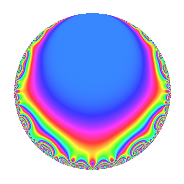Properties

 Label 945.2.a.bLevel 945 Weight 2 Character orbit 945.a Self dual Yes Analytic conductor 7.546 Analytic rank 0 Dimension 2 CM No Inner twists 1

Related objects

Newspace parameters

 Level: $$N$$ = $$945 = 3^{3} \cdot 5 \cdot 7$$ Weight: $$k$$ = $$2$$ Character orbit: $$[\chi]$$ = 945.a (trivial)

Newform invariants

 Self dual: Yes Analytic conductor: $$7.54586299101$$ Analytic rank: $$0$$ Dimension: $$2$$ Coefficient field: $$\Q(\sqrt{2})$$ Coefficient ring: $$\Z[a_1, a_2]$$ Coefficient ring index: $$1$$ Fricke sign: $$-1$$ Sato-Tate group: $\mathrm{SU}(2)$

$q$-expansion

Coefficients of the $$q$$-expansion are expressed in terms of $$\beta = \sqrt{2}$$. We also show the integral $$q$$-expansion of the trace form.

 $$f(q)$$ $$=$$ $$q$$ $$+ ( -1 + \beta ) q^{2}$$ $$+ ( 1 - 2 \beta ) q^{4}$$ $$- q^{5}$$ $$- q^{7}$$ $$+ ( -3 + \beta ) q^{8}$$ $$+O(q^{10})$$ $$q$$ $$+ ( -1 + \beta ) q^{2}$$ $$+ ( 1 - 2 \beta ) q^{4}$$ $$- q^{5}$$ $$- q^{7}$$ $$+ ( -3 + \beta ) q^{8}$$ $$+ ( 1 - \beta ) q^{10}$$ $$+ ( 1 + \beta ) q^{11}$$ $$+ ( 1 - \beta ) q^{13}$$ $$+ ( 1 - \beta ) q^{14}$$ $$+ 3 q^{16}$$ $$+ ( -2 + 2 \beta ) q^{17}$$ $$+ ( 3 + \beta ) q^{19}$$ $$+ ( -1 + 2 \beta ) q^{20}$$ $$+ q^{22}$$ $$+ ( -2 - 2 \beta ) q^{23}$$ $$+ q^{25}$$ $$+ ( -3 + 2 \beta ) q^{26}$$ $$+ ( -1 + 2 \beta ) q^{28}$$ $$+ 4 q^{29}$$ $$+ 6 q^{31}$$ $$+ ( 3 + \beta ) q^{32}$$ $$+ ( 6 - 4 \beta ) q^{34}$$ $$+ q^{35}$$ $$+ 6 \beta q^{37}$$ $$+ ( -1 + 2 \beta ) q^{38}$$ $$+ ( 3 - \beta ) q^{40}$$ $$+ ( 5 - 2 \beta ) q^{41}$$ $$+ ( -1 + 4 \beta ) q^{43}$$ $$+ ( -3 - \beta ) q^{44}$$ $$-2 q^{46}$$ $$+ ( 1 + 6 \beta ) q^{47}$$ $$+ q^{49}$$ $$+ ( -1 + \beta ) q^{50}$$ $$+ ( 5 - 3 \beta ) q^{52}$$ $$+ ( 1 - 7 \beta ) q^{53}$$ $$+ ( -1 - \beta ) q^{55}$$ $$+ ( 3 - \beta ) q^{56}$$ $$+ ( -4 + 4 \beta ) q^{58}$$ $$+ ( -2 - 6 \beta ) q^{59}$$ $$+ 6 \beta q^{61}$$ $$+ ( -6 + 6 \beta ) q^{62}$$ $$+ ( -7 + 2 \beta ) q^{64}$$ $$+ ( -1 + \beta ) q^{65}$$ $$+ ( 5 - 2 \beta ) q^{67}$$ $$+ ( -10 + 6 \beta ) q^{68}$$ $$+ ( -1 + \beta ) q^{70}$$ $$-2 q^{71}$$ $$+ ( 5 + 5 \beta ) q^{73}$$ $$+ ( 12 - 6 \beta ) q^{74}$$ $$+ ( -1 - 5 \beta ) q^{76}$$ $$+ ( -1 - \beta ) q^{77}$$ $$+ ( 12 - 2 \beta ) q^{79}$$ $$-3 q^{80}$$ $$+ ( -9 + 7 \beta ) q^{82}$$ $$+ ( -1 - 6 \beta ) q^{83}$$ $$+ ( 2 - 2 \beta ) q^{85}$$ $$+ ( 9 - 5 \beta ) q^{86}$$ $$+ ( -1 - 2 \beta ) q^{88}$$ $$+ ( 3 - 4 \beta ) q^{89}$$ $$+ ( -1 + \beta ) q^{91}$$ $$+ ( 6 + 2 \beta ) q^{92}$$ $$+ ( 11 - 5 \beta ) q^{94}$$ $$+ ( -3 - \beta ) q^{95}$$ $$-10 \beta q^{97}$$ $$+ ( -1 + \beta ) q^{98}$$ $$+O(q^{100})$$ $$\operatorname{Tr}(f)(q)$$ $$=$$ $$2q$$ $$\mathstrut -\mathstrut 2q^{2}$$ $$\mathstrut +\mathstrut 2q^{4}$$ $$\mathstrut -\mathstrut 2q^{5}$$ $$\mathstrut -\mathstrut 2q^{7}$$ $$\mathstrut -\mathstrut 6q^{8}$$ $$\mathstrut +\mathstrut O(q^{10})$$ $$2q$$ $$\mathstrut -\mathstrut 2q^{2}$$ $$\mathstrut +\mathstrut 2q^{4}$$ $$\mathstrut -\mathstrut 2q^{5}$$ $$\mathstrut -\mathstrut 2q^{7}$$ $$\mathstrut -\mathstrut 6q^{8}$$ $$\mathstrut +\mathstrut 2q^{10}$$ $$\mathstrut +\mathstrut 2q^{11}$$ $$\mathstrut +\mathstrut 2q^{13}$$ $$\mathstrut +\mathstrut 2q^{14}$$ $$\mathstrut +\mathstrut 6q^{16}$$ $$\mathstrut -\mathstrut 4q^{17}$$ $$\mathstrut +\mathstrut 6q^{19}$$ $$\mathstrut -\mathstrut 2q^{20}$$ $$\mathstrut +\mathstrut 2q^{22}$$ $$\mathstrut -\mathstrut 4q^{23}$$ $$\mathstrut +\mathstrut 2q^{25}$$ $$\mathstrut -\mathstrut 6q^{26}$$ $$\mathstrut -\mathstrut 2q^{28}$$ $$\mathstrut +\mathstrut 8q^{29}$$ $$\mathstrut +\mathstrut 12q^{31}$$ $$\mathstrut +\mathstrut 6q^{32}$$ $$\mathstrut +\mathstrut 12q^{34}$$ $$\mathstrut +\mathstrut 2q^{35}$$ $$\mathstrut -\mathstrut 2q^{38}$$ $$\mathstrut +\mathstrut 6q^{40}$$ $$\mathstrut +\mathstrut 10q^{41}$$ $$\mathstrut -\mathstrut 2q^{43}$$ $$\mathstrut -\mathstrut 6q^{44}$$ $$\mathstrut -\mathstrut 4q^{46}$$ $$\mathstrut +\mathstrut 2q^{47}$$ $$\mathstrut +\mathstrut 2q^{49}$$ $$\mathstrut -\mathstrut 2q^{50}$$ $$\mathstrut +\mathstrut 10q^{52}$$ $$\mathstrut +\mathstrut 2q^{53}$$ $$\mathstrut -\mathstrut 2q^{55}$$ $$\mathstrut +\mathstrut 6q^{56}$$ $$\mathstrut -\mathstrut 8q^{58}$$ $$\mathstrut -\mathstrut 4q^{59}$$ $$\mathstrut -\mathstrut 12q^{62}$$ $$\mathstrut -\mathstrut 14q^{64}$$ $$\mathstrut -\mathstrut 2q^{65}$$ $$\mathstrut +\mathstrut 10q^{67}$$ $$\mathstrut -\mathstrut 20q^{68}$$ $$\mathstrut -\mathstrut 2q^{70}$$ $$\mathstrut -\mathstrut 4q^{71}$$ $$\mathstrut +\mathstrut 10q^{73}$$ $$\mathstrut +\mathstrut 24q^{74}$$ $$\mathstrut -\mathstrut 2q^{76}$$ $$\mathstrut -\mathstrut 2q^{77}$$ $$\mathstrut +\mathstrut 24q^{79}$$ $$\mathstrut -\mathstrut 6q^{80}$$ $$\mathstrut -\mathstrut 18q^{82}$$ $$\mathstrut -\mathstrut 2q^{83}$$ $$\mathstrut +\mathstrut 4q^{85}$$ $$\mathstrut +\mathstrut 18q^{86}$$ $$\mathstrut -\mathstrut 2q^{88}$$ $$\mathstrut +\mathstrut 6q^{89}$$ $$\mathstrut -\mathstrut 2q^{91}$$ $$\mathstrut +\mathstrut 12q^{92}$$ $$\mathstrut +\mathstrut 22q^{94}$$ $$\mathstrut -\mathstrut 6q^{95}$$ $$\mathstrut -\mathstrut 2q^{98}$$ $$\mathstrut +\mathstrut O(q^{100})$$

Embeddings

For each embedding $$\iota_m$$ of the coefficient field, the values $$\iota_m(a_n)$$ are shown below.

For more information on an embedded modular form you can click on its label.

Label $$\iota_m(\nu)$$ $$a_{2}$$ $$a_{3}$$ $$a_{4}$$ $$a_{5}$$ $$a_{6}$$ $$a_{7}$$ $$a_{8}$$ $$a_{9}$$ $$a_{10}$$
1.1
 −1.41421 1.41421
−2.41421 0 3.82843 −1.00000 0 −1.00000 −4.41421 0 2.41421
1.2 0.414214 0 −1.82843 −1.00000 0 −1.00000 −1.58579 0 −0.414214
 $$n$$: e.g. 2-40 or 990-1000 Significant digits: Format: Complex embeddings Normalized embeddings Satake parameters Satake angles

Inner twists

This newform does not admit any (nontrivial) inner twists.

Atkin-Lehner signs

$$p$$ Sign
$$3$$ $$-1$$
$$5$$ $$1$$
$$7$$ $$1$$

Hecke kernels

This newform can be constructed as the intersection of the kernels of the following linear operators acting on $$S_{2}^{\mathrm{new}}(\Gamma_0(945))$$:

 $$T_{2}^{2}$$ $$\mathstrut +\mathstrut 2 T_{2}$$ $$\mathstrut -\mathstrut 1$$ $$T_{11}^{2}$$ $$\mathstrut -\mathstrut 2 T_{11}$$ $$\mathstrut -\mathstrut 1$$